The HPLOGISTIC Procedure

PROC HPLOGISTIC Statement

• PROC HPLOGISTIC <options>;

The PROC HPLOGISTIC statement invokes the procedure. Table 10.1 summarizes the available options in the PROC HPLOGISTIC statement by function. The options are then described fully in alphabetical order.

Table 10.1: PROC HPLOGISTIC Statement Options

Option

Description

Basic Options

Specifies a global significance level

Specifies the input data set

Limits the length of effect names

Options Related to Output

Adds detail information to "Iteration History" table

Displays the "Iteration History" table with model selection

Suppresses ODS output

Limits or suppresses the display of class levels

Suppresses generation of the iteration history table

Suppresses computation of covariance matrix and standard errors

Options Related to Optimization

Tunes the absolute function convergence criterion

Tunes the absolute function difference convergence criterion

Tunes the absolute gradient convergence criterion

Tunes the relative function difference convergence criterion

Tunes the relative gradient convergence criterion

Specifies the SAS data set that contains the starting values

Chooses the maximum number of iterations in any optimization

Specifies the maximum number of function evaluations in any optimization

Specifies the upper limit of CPU time (in seconds) for any optimization

Specifies the minimum number of iterations in any optimization

Specifies whether the objective function is normalized during optimization

Adds parameter name to the "Parameter Estimates" table

Selects the optimization technique

Tolerances

Tunes the singularity criterion for Cholesky decompositions

Tunes the singularity criterion for the sweep operator

Tunes the general singularity criterion

User-Defined Formats

Specifies the file reference for a format stream

You can specify the following options in the PROC HPLOGISTIC statement.

ABSCONV=r
ABSTOL=r

specifies an absolute function convergence criterion. For minimization, termination requiresr, where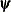is the vector of parameters in the optimization and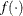is the objective function. The default value of r is the negative square root of the largest double-precision value, which serves only as a protection against overflows.

ABSFCONV=r <n>
ABSFTOL=r <n>

specifies an absolute function difference convergence criterion. For all techniques except NMSIMP, termination requires a small change of the function value in successive iterations: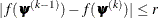Here,denotes the vector of parameters that participate in the optimization, andis the objective function. The same formula is used for the NMSIMP technique, but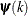is defined as the vertex with the lowest function value and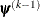is defined as the vertex with the highest function value in the simplex. The default value is r = 0. The optional integer value n specifies the number of successive iterations for which the criterion must be satisfied before the process can be terminated.

ABSGCONV=r <n>
ABSGTOL=r <n>

specifies an absolute gradient convergence criterion. Termination requires the maximum absolute gradient element to be small: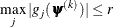Here,denotes the vector of parameters that participate in the optimization, and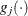is the gradient of the objective function with respect to the jth parameter. This criterion is not used by the NMSIMP technique. The default value is r=1E–5. The optional integer value n specifies the number of successive iterations for which the criterion must be satisfied before the process can be terminated.

ALPHA=number

specifies a global significance level for the construction of confidence intervals. The confidence level is 1–number. The value of number must be between 0 and 1; the default is 0.05. You can override the global specification with the ALPHA= option in the MODEL statement.

DATA=SAS-data-set

names the input SAS data set for PROC HPLOGISTIC to use. The default is the most recently created data set.

If the procedure executes in distributed mode, the input data are distributed to memory on the appliance nodes and analyzed in parallel, unless the data are already distributed in the appliance database. In that case the procedure reads the data alongside the distributed database. For information about the various execution modes, see the section Processing Modes; for information about the alongside-the-database model, see the section Alongside-the-Database Execution.

FCONV=r <n>
FTOL=r <n>

specifies a relative function difference convergence criterion. For all techniques except NMSIMP, termination requires a small relative change of the function value in successive iterations,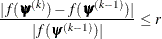Here,denotes the vector of parameters that participate in the optimization, andis the objective function. The same formula is used for the NMSIMP technique, but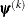is defined as the vertex with the lowest function value, andis defined as the vertex with the highest function value in the simplex.

The default value is r=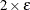whereis the machine precision. The optional integer value n specifies the number of successive iterations for which the criterion must be satisfied before the process can terminate.

FMTLIBXML=file-ref

specifies the file reference for the XML stream that contains the user-defined format definitions. User-defined formats are handled differently in a distributed computing environment than they are in other SAS products. For more information about how to generate a XML stream for your formats, see the section Working with Formats.

GCONV=r <n>
GTOL=r <n>

specifies a relative gradient convergence criterion. For all techniques except CONGRA and NMSIMP, termination requires that the normalized predicted function reduction be small,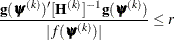Here,denotes the vector of parameters that participate in the optimization,is the objective function, and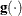is the gradient. For the CONGRA technique (where a reliable Hessian estimateis not available), the following criterion is used: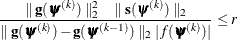This criterion is not used by the NMSIMP technique. The default value is r=1E–8. The optional integer value n specifies the number of successive iterations for which the criterion must be satisfied before the process can terminate.

INEST=SAS-data-set

names the TYPE=EST SAS data set that contains starting values for the parameters.

Your data set must include the _TYPE_ variable, a character variable in which the value 'PARMS' indicates the observation that contains your starting values. The data set also includes a numeric variable for each parameter for which you are specifying a starting value; the name of this numeric variable is the "parameter name." You can obtain parameter names by specifying the OUTEST option and by using the ODS OUTPUT statement to output the "Parameter Estimates" table into a data set; the parameter name is contained in the ParmName variable in this data set. If you do not specify a starting value for a parameter, it is set to 0. PROC HPLOGISTIC uses only the first observation for which _TYPE_=PARMS, and it ignores BY variables. For more information about TYPE=EST data sets, see Appendix A: Special SAS Data Sets in SAS/STAT 14.1 User's Guide.

If you specify TECH=NONE or MAXITER=0, then the values in the INEST= data set are used as the parameter estimates, but the null model is still computed at the optimum value for the intercepts. If you specify TECH=NONE or MAXITER=0 and you specify a null model in the MODEL statement, then the null model is computed at the starting values for the intercept parameters.

ITDETAILS

adds to the "Iteration History" table the current values of the parameter estimates and their gradients. These quantities are reported only for parameters that participate in the optimization.

ITSELECT

generates the "Iteration History" table when you perform a model selection.

MAXFUNC=n
MAXFU=n

specifies the maximum number n of function calls in the optimization process. The default values are as follows, depending on the optimization technique:

• TRUREG, NRRIDG, NEWRAP: 125

• QUANEW, DBLDOG: 500

• CONGRA: 1,000

• NMSIMP: 3,000

The optimization can terminate only after completing a full iteration. Therefore, the number of function calls that are actually performed can exceed the number that is specified by the MAXFUNC= option. You can choose the optimization technique with the TECHNIQUE= option.

MAXITER=n
MAXIT=n

specifies the maximum number n of iterations in the optimization process. The default values are as follows, depending on the optimization technique:

• TRUREG, NRRIDG, NEWRAP: 50

• QUANEW, DBLDOG: 200

• CONGRA: 400

• NMSIMP: 1,000

These default values also apply when n is specified as a missing value. You can choose the optimization technique with the TECHNIQUE= option.

MAXTIME=r

specifies an upper limit of r seconds of CPU time for the optimization process. The default value is the largest floating-point double representation of your computer. The time specified by the MAXTIME= option is checked only once at the end of each iteration. Therefore, the actual running time can be longer than that specified by the MAXTIME= option.

MINITER=n
MINIT=n

specifies the minimum number of iterations. The default value is 0. If you request more iterations than are actually needed for convergence to a stationary point, the optimization algorithms can behave strangely. For example, the effect of rounding errors can prevent the algorithm from continuing for the required number of iterations.

NAMELEN=number

specifies the length to which long effect names are shortened. The default and minimum value is 20.

NOCLPRINT<=number>

suppresses the display of the "Class Level Information" table if you do not specify number. If you specify number, the values of the classification variables are displayed for only those variables whose number of levels is less than number. Specifying a number helps to reduce the size of the "Class Level Information" table if some classification variables have a large number of levels.

NOITPRINT

suppresses the generation of the "Iteration History" table.

NOPRINT

suppresses the generation of ODS output.

NORMALIZE=YES | NO

specifies whether the objective function should be normalized during the optimization by the reciprocal of the used frequency count. The default is to normalize the objective function. This option affects the values reported in the "Iteration History" table. The results reported in the "Fit Statistics" are always displayed for the nonnormalized log-likelihood function.

NOSTDERR

suppresses the computation of the covariance matrix and the standard errors of the logistic regression coefficients. When the model contains many variables (thousands), the inversion of the Hessian matrix to derive the covariance matrix and the standard errors of the regression coefficients can be time-consuming.

OUTEST

adds a column for the ParmName variable to the "Parameter Estimates" table. This column is not displayed, but you can use it to create a data set that you can specify in an INEST= option by first using the ODS OUTPUT statement to output the "Parameter Estimates" table and then submitting the following statements:

proc transpose data=parameterestimates out=inest(type=EST) label=_TYPE_;
label Estimate=PARMS;
var Estimate;
id ParmName;
run;

SINGCHOL=number

tunes the singularity criterion in Cholesky decompositions. The default is 1E7 times the machine epsilon; this product is approximately 1E–9 on most computers.

SINGSWEEP=number

tunes the singularity criterion for sweep operations. The default is 1E7 times the machine epsilon; this product is approximately 1E–9 on most computers.

SINGULAR=number

tunes the general singularity criterion applied by the HPLOGISTIC procedure in sweeps and inversions. The default is 1E7 times the machine epsilon; this product is approximately 1E–9 on most computers.

TECHNIQUE=keyword
TECH=keyword

specifies the optimization technique for obtaining maximum likelihood estimates. You can choose from the following techniques by specifying the appropriate keyword:

CONGRA

DBLDOG

performs a version of double-dogleg optimization.

NEWRAP

performs a Newton-Raphson optimization with line search.

NMSIMP

NONE

performs no optimization.

NRRIDG

performs a Newton-Raphson optimization with ridging.

QUANEW

performs a dual quasi-Newton optimization.

TRUREG

performs a trust-region optimization

The default value is TECHNIQUE=NRRIDG.